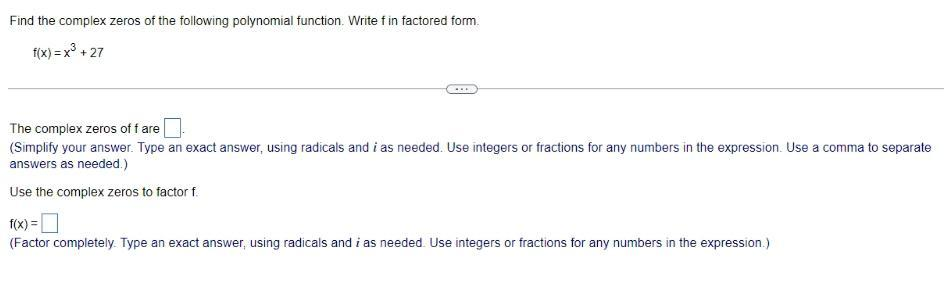Question:

# Find the complex zeros of the following polynomial function.

Last updated: 8/14/2022Find the complex zeros of the following polynomial function. Write f in factored form. f(x)=x³ +27 The complex zeros of f are Use the complex zeros to factor f f(x) =